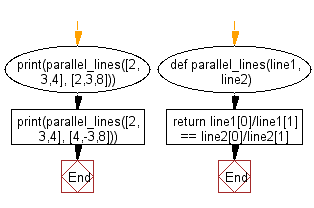﻿ Python: Check whether two given lines are parallel or not - w3resource# Python: Check whether two given lines are parallel or not

## Python Basic - 1: Exercise-103 with Solution

Write a Python program to check whether two given lines are parallel or not.
Note: Parallel lines are two or more lines that never intersect. Parallel Lines are like railroad tracks that never intersect.
The General Form of the equation of a straight line is: ax + by = c
The said straight line is represented in a list as [a, b, c]
Example of two parallel lines:
x + 4y = 10 and x + 4y = 14

Sample Solution:

Python Code:

``````def parallel_lines(line1, line2):
return line1/line1 == line2/line2
#2x + 3y = 4, 2x + 3y = 8
print(parallel_lines([2,3,4], [2,3,8]))
#2x + 3y = 4, 4x - 3y = 8
print(parallel_lines([2,3,4], [4,-3,8]))
``````

Sample Output:

```True
False
```

Flowchart:Python Code Editor:

Have another way to solve this solution? Contribute your code (and comments) through Disqus.

What is the difficulty level of this exercise?

Test your Programming skills with w3resource's quiz.

﻿

## Python: Tips of the Day

Iterating over dictionaries using 'for' loops:

I am a bit puzzled by the following code: d = {'x': 1, 'y': 2, 'z': 3} for key in d: print key, 'corresponds to', d[key] What I don't understand is the key portion. How does Python recognize ...

key is just a variable name.

```for key in d:
```

For Python 3.x:

```for key, value in d.items():
```

For Python 2.x:

```for key, value in d.iteritems():
```

To test for yourself, change the word key to poop.

In Python 3.x, iteritems() was replaced with simply items(), which returns a set-like view backed by the dict, like iteritems() but even better. This is also available in 2.7 as viewitems().

The operation items() will work for both 2 and 3, but in 2 it will return a list of the dictionary's (key, value) pairs, which will not reflect changes to the dict that happen after the items() call. If you want the 2.x behavior in 3.x, you can call list(d.items()).

Ref: https://bit.ly/37dm0Qo